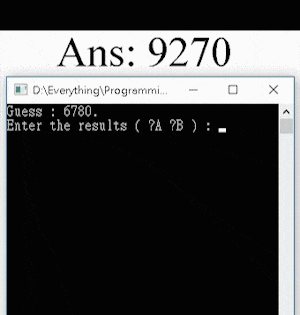# Guess the Number AI

After completing the first two projects of the Guess the Number series (Guess the Number (Windows) and Guess the Number (iOS)), I made an AI that can play this game at a high-human level.

It has been proven that at most 7 turns are needed to guess the answer, with a best average game length of 5.21 turns. For this game, all the possible combinations (e.g. “0123”, “7381” …) can be saved into a 1D array. After each guess, the possible combinations for the answer will be reduced. Therefore, the algorithm of the program is written for finding a number that will minimize the maximum possible combinations left. The time complexity for each turn is O(n3), and an average of 5 turns of guessing if needed for an arbitrarily chosen number.

For using the program, the user must first choose a 4-digit answer (e.g. “0123”), and input the two numbers [A] and [B] according to the game rules and the numbers guessed by the program. For instance, if the answer is “1357”, and the AI guesses “3127”, the user must input 1 2 ([A] = 1, [B] = 2).

Here’s a demonstration of the AI program guessing the answer “8192” in 5 guesses: